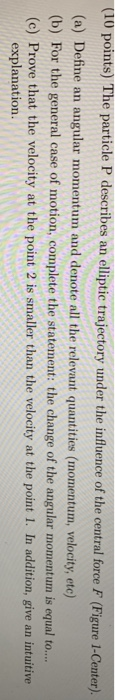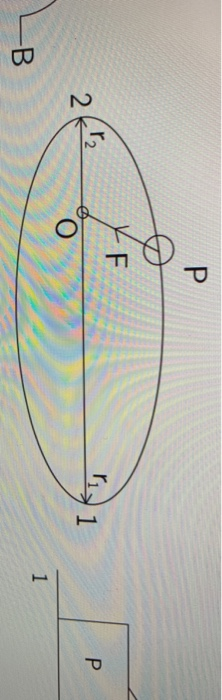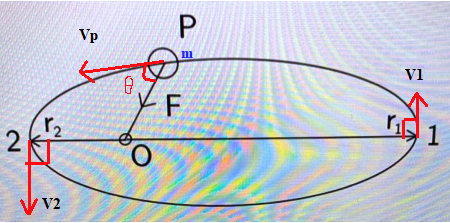Homework Help Question & Answers

# (10 points) The particle P descr ibes an elliptic trajectory under the influence of the central force F (Figure 1-Center). (a) Define an angular momentum and denote all the relevant quantities (...(10 points) The particle P descr ibes an elliptic trajectory under the influence of the central force F (Figure 1-Center). (a) Define an angular momentum and denote all the relevant quantities (momentum, velocity, etc) (b) For the general case of motion, complete the statement: the change of the angular momentum is equal to.. (c) Prove that the velocity at the point 2 is smaller than the velocity at the point 1. In addition, give an intuitive explanation
1 1 2 2

Solution: Here we are given a particle moving in an elliptical trajectory under the influence of a central force, which is directed towards the center of rotation O.

(a)

• Angular momentum is a vector quantity which is used as a measure of the rotational momentum of a rotating body or system, that is equal in classical physics to the product of the angular velocity of the body or system and its moment of inertia with respect to the rotation axis, and that is directed along the rotation axis.
• Or, we can define the angular momentum as the vector cross product of the position vector of the particle and its linear momentum which is also a vector.• For a particle of mass m, moving with a certain velocity,at a certain position,with respect to the axis of rotation, O, the angular momentum,is defined as:whereis the linear momentum of the particle, which is defined as the momentum of translation, being a vector quantity in classical physics, equal to the product of the mass and the velocity of the center of mass. It is given as -• Putting this value in the equation for angular momentum, we haveAnd its magnitude is given bywhereis the angle betweenand.

(b) For a general case of motion:

• "The time rate of change of angular momentum of a particle is equal to the vector sum of all torques acting on the particle."

(c)

• The motion of any particle in such an elliptical trajectory is only possible when it is subjected to a conservative force which is acting towards the axis of rotation. Under such force, the energy and the angular momentum of such a particle are always conserved. One example of such a force is the force of gravity acting between earth and the sun where sun orbits around the sun in a similar elliptical path.
• So, applying the conservation of angular momentum of the particle in positions 1 and 2, we getwhere r1 and r2 are the apogee and perigee of the elliptical path andSo,Since##### Add Answer of: (10 points) The particle P descr ibes an elliptic trajectory under the influence of the central force F (Figure 1-Center). (a) Define an angular momentum and denote all the relevant quantities (...
More Homework Help Questions Additional questions in this topic.

• #### Physics problem particle under influence of force

Need Online Homework Help?

Get FREE EXPERT Answers
WITHIN MINUTES
Related Questions